# Large Eddy Simulation of the PVC Behavior in both Non-Reacting and Reacting Flows with Different Reynold Numbers

Large Eddy Simulation of the PVC Behavior in both Non-Reacting and Reacting Flows with Different Reynold Numbers

Shi LiFu Zhongguang Shen Yazhou Wang Ruixin Zhang Hui

School of Energy, Power and Mechanical Engineering, North China Electric Power University, Beijing 102206, PR China

Corresponding Author Email:
hnulee@sina.com
Page:
429-438
|
DOI:
https://doi.org/10.18280/ijht.340312
|
Accepted:
|
Published:
30 September 2016
| Citation

OPEN ACCESS

Abstract:

The effects of the Reynold number on the precessing vortex core (PVC) behavior in both non-reacting and reacting flows are numerically studied using a three-dimensional fully compressible large eddy simulation method. The predicted results show that a central toroidal recirculation zone (CTRZ) is generated in the vicinity of the axis of the combustor which is dominated by many small-scale vortices. Meanwhile, two smaller external recirculation zones (ERZs) are established in the vicinity of the sidewall of the combustion chamber. Under combustion conditions, the flow field tends to become complex, and the structure of CTRZ is more irregular compared to that of the non-reacting flow. An increase in the inlet Reynold number enlarges the magnitude of CTRZ and thus produces a more regular and stronger structure of the PVC inside the combustion chamber. Consequently, the breakdown positon of the PVC moves downstream under higher Reynold numbers. The structure of PVCs in the reacting flow mostly located in the regions of the unburned reactants within the per-chamber which is relatively smaller and irregular compared to that in the non-reacting flow. There is a linear increase in pressure oscillations amplitude and frequency with an increase in the inlet Reynold numbers. However, the Strouhal number remains relatively constant in both non-reacting and reacting flows. There is also an anticipated decrease in pressure oscillations and frequency in the reacting flow due to the compressibility effect in the reacting flow.

Keywords:

Large eddy simulation, Reynold number, Recirculation zone, Precessing vortex core.

1. Introduction

To improve the combustion stabilization of lean-premixed flame, swirling flows has been widely used in the gas turbine combustion systems [1, 2]. In high swirl flow conditions, the breakdown of vortex induces the formation of central toroidal recirculation zone (CTRZ) inside the combustion chamber . As a result, the heat produced in the combustion recirculate upstream to the exit of the combustor thus improves the stabilization of the premixed-flame [3, 4]. Bubble type, axisymmetric with asymmetric tail, spiral, flattened bubble, flattened bubble spiral, double helix and sheared filament type are the basic type of the vortex core breakdown modes according to the studies of Leibovich et al.  and Faler et al. . The type of vortex core breakdown modes are closely related to Reynold number (Re) and swirl number (Sg) [7, 8]. As research continues, the phenomenon of vortex breakdown in laminar flows with low Reynold numbers are also observed in some other studies [9, 10].

Vortex breakdown can be asymmetric as well as highly time dependent [1, 2]. A precessing vortex core (PVC) develops when the vortex cores precess around the center axis and thus produces an undesired low-frequency acoustic oscillation inside the combustion chamber. The exit of PVC may trigger the combustion instabilities which is harmful to the safe operation of the unit and structure of the combustion chamber . Furthermore, the oscillation frequency of the PVC is closely related to combustor geometry, Reynold number and swirl number in the non-reacting flow. Guo et al.  numerically studied the combustion instabilities in swirl stabilized flames and found that the PVC tends to occur in lower swirl numbers for a combustor with an expanded section or central bluff body. Kilian et al.  studied the impacts of Reynold number on the flow field in a GT model combustor under atmospheric pressure conditions and found that the frequency of the PVC increases linearly with an increase in the inlet Reynold number of premixed air and methane in non-reacting flow. Similar research conclusions of inlet Reynold number or volumetric flow rate on instability frequency in non-reacting flow also can be found in some other related studies . Furthermore, the occurrence of pressure is relatively more complex in the reacting flow . In some studies, the PVCs occurs in the GT combustion chamber in both non-reacting and reacting flows, and the structure of the PVC and associated flows in the reacting flow is similar to that in the non-reacting flow . However, the PVC only occurs in the non-reacting flow, and can be suppressed in the reacting flow in other studies . The frequency of pressure occurrence is closely related to the preheat temperature, Reynold number and swirl number, pilot fuel distribution . Bulat et al.  numerically studied the fluid dynamics inside the combustor chamber and found that the coherence of the PVC structure is relatively stronger in the non-reacting flow due to compressibility effects of the flame in the reacting flow. Syred et al.  numerically studied the effect of the Reynold number and equivalence ratio upon the Strouhal number (St) and concluded that the PVC as a function of the Reynold number and equivalence and the values of Strouhal number in the reacting flow approaches 80–90 % of that in the non-reacting flow. Similar research conclusions also can be found in other studies . In some other studies, the PVC may trigger the combustion instabilities and the frequency of occurrences of pressure in the reacting flow. Kilian et al.  experimentally studied the flame and flow field in a GT model combustor via simultaneous high-speed PIV and OH-PLIF in a reacting flow and found that the PVC only happens in an M-shape flame. The frequencies of the PVC in a reacting flow are slightly higher than that of the non-reacting flows. Therefore, there is no consensus on the effects of the inlet Reynolds number on the behavior of the PVC both in non-reacting and reacting flows inside the combustion chamber.

Reynolds Average Navier-Stokes (RANS) approach which developed for mean stationary flows is widely used in cases which do not require high precision . Unsteady computing and modeling strategies which take into account unsteadiness and in-homogeneities are alternative numerical solutions to further improve the numerical calculation precision . It is noted that great advances in the development of Direct Numerical Simulations (DNS) and Large Eddy Simulations (LES) have occurred due to the large improvement in computing power and the rise of massively parallel architectures . Up to present, the DNS approach still has difficulty dealing with the aeronautical reactive flows due to the large computational resource needed, especially in high Reynold number turbulence flow . Compared with the other two approaches, only large motions are directly resolved while the small scale motions are modeled in the LES approach . Therefore, four dimensional time and space parameters of flow and chemical thermodynamics field can be easily accessed by using the LES approach at relatively smaller calculation time . Furthermore, it is easy to study the behavior of the PVC inside the GT combustion chamber which is the main reason for the combustion instability. In this study, six LES test cases are selected to investigate the influence of the inlet Reynold on the PVC behavior both in non-reacting and reacting flows which are 88 500, 118 000 and 147 500. The inlet Reynold are varied by changing the flow rate of incoming fuel/oxidant streams. Case E represents the reference case with inlet Reynold number of 118 000 in the reacting flow. Before analyzing, the LES results of case E is validated with experiments results . Relationships between Reynold number, axial velocity, flame temperature and the PVC behavior both in non-reacting and reacting flows will be discussed in detail in sections 3.2-3.4.

2. Numerical Simulations

2.1 Model combustion chamber

## figure_1.png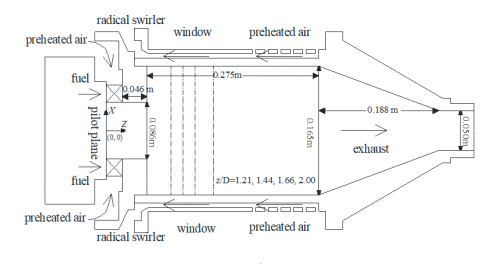Figure 1. Cross section of the model GT combustion chamber and DLE combustor

2.2 Numerical Simulations

A parallel LES solver is applied to solve the fully compressible Navier-Stokes equations on the structured grids of the computational domain . A Favre filter is applied to the conservation equations of mass, momentum and energy yields . Separation of the large and small scale energetic motions is achieved as follows:

$\phi=\overline{\phi}+\phi^{\prime}$(1)

$\overline{\phi}=\int_{D} \phi G\left(x, x^{\prime}\right) d x^{\prime}$(2)

where $\overline{\phi}$ and $\phi^{\prime}$ are the large scale solvable variables and small scale unsolvable variables respectively. D is the flow region and $G\left(x, x^{\prime}\right)$ is the density weighted filter function.

Mass, momentum and energy are determined as follows:

$\frac{\partial \overline{\rho}}{\partial t}+\frac{\partial\left(\overline{\rho} \overline{u}_{i}\right)}{\partial x_{i}}=0$(3)

$\frac{\partial\left(\overline{\rho u}_{i}\right)}{\partial t}+\frac{\partial\left(\overline{\rho} \widetilde{u}_{i} \widetilde{u}_{j}+\overline{p} \delta_{i j}\right)}{\partial x_{j}}=\frac{\partial\left(\overline{\tau}_{i j}+\overline{\tau}_{i j}^{s g s}\right)}{\partial x_{j}}$(4)

$\frac{\partial(\overline{\rho} \overline{E})}{\partial t}+\frac{\partial\left((\overline{\rho} \overline{E}+\overline{p}) \overline{u}_{i}\right)}{\partial x_{j}}=\frac{\partial}{\partial x_{i}}\left(\overline{u}_{j} \overline{\tau}_{i j}+\lambda \frac{\partial \overline{T}}{\partial x_{i}}-H_{i}^{s g s}+\sigma_{i}^{s g s}\right)$(5)

where  $\tau_{i j}^{s g s}, \quad H_{i}^{s g s} \quad$ and $\quad \sigma_{i}^{s g s}$ are the sub-grid scale stress tensor, sub-grid scale flux of energy and the sub-grid viscous diffusion term respectively . Variables filtering by spatial-filtering operation are denoted by the overbars (-) such as pressure and density while variables filtering by Favre-filtering operation are denoted by a tilde (~) such as velocity, temperature and enthalpy.

$\tau_{i j}^{s g s}=-2 \mu_{s} \overline{S}_{i j}+1 / 3 \tau_{k k} \delta_{i j}$(6)

$H_{i}^{s g s}=-\frac{\overline{\rho} v_{t}}{\operatorname{Pr}_{t}} \frac{\partial H}{\partial x}$(7)

Laminar Finite Rate, Finite Rate /Eddy Dissipation and Eddy Dissipation Concept model are the sub-grid scale combustion models provided in FLUENT code [1, 2]. The Finite Rate /Eddy Dissipation model are used in this study, which uses the smaller one of Arrhenius and eddy dissipation rate as the net reaction rate [1, 2]. Expressions of Arrhenius and eddy dissipation rates in the Finite Rate /Eddy Dissipation model are determined as follows:

$R_{i, r}=v_{i, r}^{\prime} M_{w, r} A \rho \frac{\varepsilon}{k} \min _{R}\left(\frac{Y_{R}}{v_{R, r}^{\prime} M_{w, R}}\right)$(8)

$R_{i, r}=v_{i, r}^{\prime} M_{w, r} A B \rho \frac{\varepsilon}{k} \frac{\sum_{p} Y_{P}}{\sum_{j}^{N} v_{j, r} M_{w, j}}$(9)

Because of the enormous computational resources required for solving all the species, a reduced M4 mechanism based on GRI-Mech 3.0 mechanisms is adopted which is consisting of four step reaction mechanisms and six species which have been verified in the studies of Bulat et al. .

$\left[C H_{4}\right]^{0.7}+1.5\left[O_{2}\right]^{0.97} \rightarrow C O+2 H_{2}$(10)

$\left[H_{2}\right]^{0.55}+0.5\left[O_{2}\right]^{101} \rightarrow H_{2} O$(11)

$[\mathrm{CO}]+0.5\left[\mathrm{O}_{2}\right]^{0.5} \rightarrow \mathrm{CO}_{2}$(12)

$[C O]+\left[H_{2} O\right] \rightarrow C O_{2}+H_{2}$(13)

The kinetic rate data of the M4 mechanism are determined as follows:

$k_{f, 1}=A_{1} T^{B 1} e^{\frac{-E_{a 1}}{R T}}\left[C H_{4}\right]^{0.7}\left[O_{2}\right]^{0.97}$(14)

$k_{f, 2}=\left(p_{a t m} / p\right)^{\mathrm{n}} A_{2} T^{B_{2}} e^{\frac{-E_{a 2}}{R T}}\left[H_{2}\right]^{0.55}\left[O_{2}\right]^{1.01}$(15)

$k_{f, 3}=A_{3} T^{B_{3}} e^{\frac{-E_{o 3}}{R T}}[C O]\left[O_{2}\right]^{0.5}$(16)

$k_{f, 4}=A_{4} T^{B 4} e^{\frac{-E_{o 4}}{R T}}[C O]\left[H_{2} O\right]$(17)

where kf is the kinetic rate, A is the pre-exponential factor, B is the temperature exponent, Ea is the activation energies, R is the ideal gas constant and T is the temperature.

## figure_2.png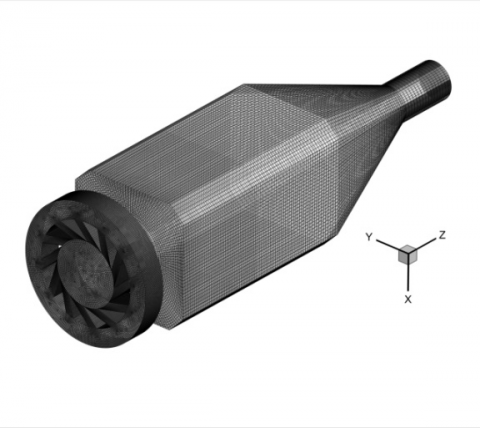Figure 2. Schematic illustration of the computaional domain and grid structure

A computational fluid dynamics (CFD) pre-processing software code Gambit is employed to establish the modeling and mesh generation in this study . Computational domain includes the model GT combustion chamber and DLE combustor (see Figure 2). Considering the complexity of the computational domain, a structured multi-grid generation method is applied to achieve high quality hexahedral mesh of the whole computational domain. A finer grid scheme is generated in the vicinity of the region to the combustor and sidewall of the combustion chamber to predict exactly the change of important coherent parameters in those regions . Considering that the LES approach still has strict requirements for the computing capability and speed of the computer and memory, a relatively larger mesh is generated in the region near the exit of the combustion chamber to control the grid number of the computer region, thus reducing the required numerical calculations . Total grid number is approximately 1 792 366. Time step is calculated by courant number rule which is kept below 0.5 during the calculation. Considering that the grid scale of the combustor region is below 1 mm, the time step is set as 1×10-6 s. In order to study the transformation of flame configuration in 0.5 s, the total number of time steps is about 250 000 for each case examined. Six LES test cases are selected to investigate the influence of the inlet Reynold number on the flow field, temperature distribution, and the PVC behavior in both non-reacting and reacting flows inside a model GT combustion chamber. Three cases with different inlet Reynold numbers are selected to estimate its effects in non-reacting flow which are 88 500, 118 000 and 147 500 respectively (cases A, B and C). Flow rates of the combustive air are 0.131 kg/s, 0.175 kg/s and 0.219 kg/s at the inlet pressure of 0.3 MPa . The setting of boundary conditions for cases D, E and F are identical to those of cases A, B and C, except that the operating conditions are varied from non-reacting to reacting flow. For cases D, E and F, the same global equivalence ratios of fuel stream are given at about 0.60 including the panel leakage air. Furthermore, the total thermal powers are 251 kW, 335 kW and 419 kW respectively . Therefore, the flow rates of the fuel are 0.131 kg/s, 0.175 kg/s and 0.219 kg/s respectively. The percentage of pilot fuel is set as 0 %. The pressure loss coefficients of the combustion chamber are set as 1 %, for all cases examined.

3. Results and Discussion

3.1 Validation of the numerical results

Figure 3 shows the comparison of LES and experimental profiles of the mean axial velocity in four different axial locations of the mid-plane for case E which is located at z/D = 1.21, 1.44, 1.66 and 2.00 from the pilot surface, where D is the diameter of the pre-chamber (see Figure 1). It is clear that the adverse pressure gradient induces a large CTRZ in the vicinity of the central axil of the combustor. The caculated axial velocity profiles show a slight asymmetry in these zones which may be improved after sufficient averaging time . The expansion of the main flow forms two peak velocities on the each side of the combustion chamber. The location and magnitude of the axial velocity and reverse flow zones are well captured at all four different axial locations although the magnitude and reverse velocity in the reverse flow zone is over-estimated than that of experimental data. Figure 4 shows the LES and experimental profiles of the mean flame temperature for the case E. The experimental data of the flame temperature only cover one-half of the combustion chamber in the studies of Stopper et al.  and Bulat et al. . It is clear that the peak flame temperature is located in the vicinity of the central axil of the combustor. Levels of temperature are relatively lower in the main flow and external recirculation zones (ERZs) of the combutison chamber. It is noted that the location and magnitude of the flame are slightly over-estimated than that of experimental data. However, the maximum error between LES and experiment results is still lower than 10 %. Similar calculation deviations can also be found in the studies of Bulat et al. . Good agreement between LES and experimental data implies that the numerical results are reasonable for studying the velocity, flame temperature distribution and the PVC behavior in both non-reacting and reacting flows.

## figure_3a.png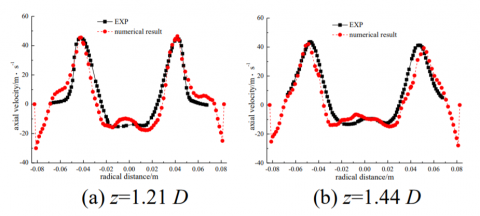## figure_3b.png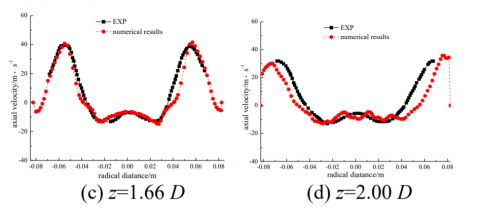Figure 3. Comparison of LES and experimental profiles of mean axial velocity (m/s)

## figure_4.pngFigure 4. Comparison of LES and experimental profiles of mean flame temperature (K)

3.2 Effect of Reynold number on flow field

Figure 5 shows the instance flow field and velocity vectors of the mid-plane close to the pre-chamber both in non-reacting and reacting flows for all cases examined. The dimension of the visualized domain is about 0.25 m, and 0.190 m in depth and width including the DLE combustor. Fresh incoming fuel/oxidant streams enter into the pre-chamber after passing through a single radial swirler mounted on the head of the DLE combustor . Under the influence of centrifugal forces, the inflow suddenly expands and thus forms an annular swirling jet inside the combustion chamber . Therefore, a CTRZ is generated in the vicinity of the axis of the combustor which is dominated by many small-scale vortices. The vortices are visualized by the plot of velocity vectors in Figure 5. The structure of CTRZ turns out to be complicated especially in the downstream region of the flowfield. The boundary of CTRZ extends to the pilot surface of the combustor which can establish a firm aerodynamic base for stabilization of the lean-premixed flame in the reacting flow . Furthermore, two smaller external recirculation zones (ERZs) are established in the vicinity of the sidewall of the chamber due to the sudden expansion of the annular swirling jet. Therefore, two shear layers are generated between the inflow, CTRZ and ERZs. The difference in the inlet Reynold number affects the location and magnitude of recirculation zones inside the combustion chamber. The velocity of inflow increases with the rising of the inlet Reynold number which increases the adverse pressure gradient and thus enlarges the magnitude and reverses the velocity in the recirculation zones in both non-reacting and reacting flows. Furthermore, the velocity of the annular swirling jet is relatively lower in the non-reacting flow. This is caused by the heat being released by the combustion reaction directly heats the inflow which decreases the density and thus improves its velocity which further enlarges the magnitude and reverse velocity in the recirculation zones. Under combustion conditions the flow field becomes more complex and diffuse. In consequence, the structure of CTRZ is more irregular compared to that of the non-reacting flow especially in the downstream of CTRZ.

## figure_5.pngFigure 5. Axial velocity distribution of the mid-plane in both non-reacting and reacting flow (m·s-1)

To understand the details of the flow field, Figures 6 and 7 shows the LES profiles of the mean axial velocity in four different axial locations of the mid-plane in both non-reacting and reacting flows for all cases examined. It is clear that the expansion of the annular swirling jet forms two symmetrical peak velocities that decrease with the increasing of the axial distance due to the diminishing of the jet inside the combustion chamber. However, the distance between the peak points is the opposite due to the expansion of the main flow. It is clear that the maximum value of the axial velocity is relatively higher in the reacting flow. At z/D= 1.21, for example, the maximum values of axial velocity increase from 40 m/s in case B (non-reacting flow) to 45 m/s in case E (reacting flow) under the same Reynold number of 118 000. The regions with zero axial velocity is usually defined as the boundary of the recirculation zones . Consequently, it is easy to compare the location and structure of recirculation zones through the profiles of the average axial velocity. It is clear that the length of CTRZ is much longer than that of ORZ which disappears when the axial distance is further than 2.00 D. Furthermore, the magnitude of the recirculation zone and the reverse velocity increase with an increase in the Reynold number in both non-reacting and reacting flows. At z/D= 1.21, for example, the breadth of CTRZ increases from 0.04 m in case A (Re= 85 000) to 0.045 m in case C (Re = 118 000).

## figure_6a.png## figure_6b.pngFigure 6. Mean axial velocity distribution along different radical locations of the mid-plane in reacting flow (m·s-1)

## figure_7.png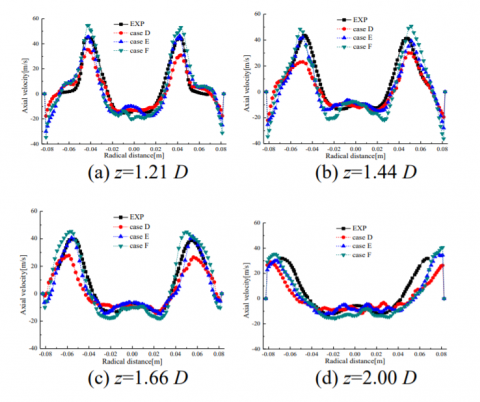Figure 7. Mean axial velocity distribution along different radical locations of the mid-plane in reacting flow (m·s-1)

3.3 Effect of Reynold number on temperature distribution

Figure 8 shows the flame temperature in the mid-plane close to the pre-chamber in the reacting flow in cases D, E and F. The gas temperature in the non-reacting flow is not included in this study due to the even distribution of temperature inside the combustion chamber. It is noted that the fresh incoming fuel/oxidant streams are numerically ignited by filling the combustion chamber with high temperature burned products which is different from the actual ignition process inside the combustion chamber . Fresh incoming premixed fuel/oxidant streams are ignited in the pre-chamber and burn downstream which formed an M-shaped flame in the shear layers between the internal and external recirculation zones. The relatively lower global equivalence compresses the premixed flame temperature inside the combustion chamber. Comparing Figure 5 with Figure 8, it is clear that the flame behavior is strongly correlate with the characteristics of the recirculation zones inside the combutsiotn chamber. A central core of hot gases and combustion products extends to the pilot surface of the combustor which can directly heat and ignite the incoming fuel/oxidant streams and thus establish a firm aerodynamic base for ignition and combustion of fuel gas. It is clear that the flame temperature is much lower near the sidewall of the combustion chamber, and a higher flame temperature persists in the vicinity of the central axis of combustor. Furthermore, the peak flame temperature increases with the increasing of the axial distance. However, the growth is subdued with the increasing of the axial distance. The thermal power and flow rate increase with the rising of the inlet Reynold number which improves the flame temperature level and enlarges the magnitude of regions with higher flame temperature inside the combustion chamber. An increase in inflow velocity also increases the stiffness of inflow and thus the extends the length of the premixed flame.

## figure_8.png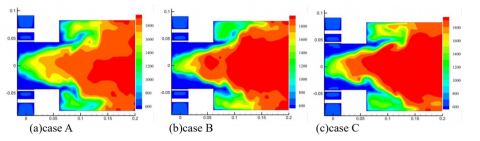Figure 8. Instance flame temperature distribution of the mid-plan in reacting flow (K)

## figure_9.png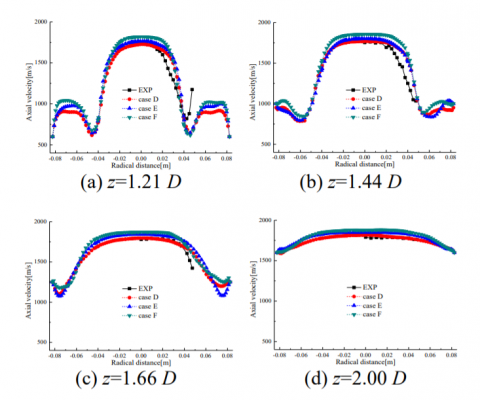Figure 9. Mean flame temperature distribution along different radical locations of the mid-plane in reacting flow (K)

Figure 9 shows the LES profiles of the mean flame temperature in the reacting flow in different axial locations of the mid-plane inr cases D, E and F. Comparing to that of the flame temperature, the average flame temperature is more symmetrical along the central axis of the combustor which indicates that the shape of the premixed flame periodically oscillates with time. The maximum values of flame temperature located in the vicinity of the central axis of the combustor, while the minimum value is in the inflow inside the combustion chamber. The flame temperature rapidly increases up to 1800 K after entering the combustion chamber. Temperature and magnitude of the flame increases with an increase in the axial distance for all cases examined. As aforementioned, the thermal powers are 251 kW, 335 kW and 419 kW for cases D, E and F respectively. It is clear that the flame temperature increases with an increase in the inlet Reynold number. At z=2.00 D, for example, the flame temperature increases from 1816 K in case D (Re=85 000) to 1878 K in case F (Re=147 500). When the flame temperature exceeds 1800 K, the increase of temperature will greatly enhance the formation process of thermal NOx according to the Zeldovich mechanisms. Therefore, the emission of NOx inside the combustion chamber relatively increases with higher inlet Reynold numbers.

3.4 Effect of Reynold number on the PVC behavior

In many previous studies, the pressure of the iso-surface is usually used to compare the structure of the PVCs. Figure 10 shows the structure of the PVCs visualized by pressure iso-surface (P=0.299 MPa), streamlines, pressure and tangential velocity in the cross section of z=0.06 m in cases A, B and C (non-reacting flow). It is clear that the PVCs with a helical structure formed in the upstream of CTRZ, in cases A, B and C. The PVC precesses in the same direction as the global rotation of the flow. Furthermore, the structure of the PVCs is anchored to the pilot surface of the combustor and persists for about 1.5–2.0 diameters of the pre-chamber before compressing into a smaller vortex as it moves downstream. In case A, there is only a single the PVC structure formed upstream of CTRZ which only persist for about 1.5 diameters of the pre-chamber as shown in Figure 10 a. Most of the liquid around the PVC structure rotates on its center. The location of the vortex overlaps with the lowest pressure region which deviates from the center axil of the combustion chamber. Furthermore, the tangential velocity in the vicinity of the PVC center is approximately zero. Therefore, the vortex has the typical feather of the PVC according to the studies of Sarpkaya et al. [7, 8]. Difference in the inlet Reynold number affects the structure of the PVC. In case B, another smaller PVC structure appears downstream of CTRZ. Furthermore, a PVC structure with approximately equal intensity occurs in case C. An increase in the Reynold number enlarges the magnitude of CTRZ and thus produces a more regular and stronger structure of the PVC inside the combustion chamber. Therefore, the breakdown positon of the PVC moves toward downstream of CTRZ with higher inlet Reynold numbers. Figure 11 shows the structure of the PVCs visualized by the pressure iso-surface (P=0.299 MPa), streamlines, pressure and tangential velocity in the cross section of z=0.06 m in cases D, E and F (reacting flow). As aforementioned, the setting of the boundary conditions for cases D, E and F are identical to that of cases A, B and C, except that the operating conditions vary from non-reacting flow to reacting flow. It is clear that the structure of the PVCs in the reacting flow are mostly in the regions of unburned reactants within the per-chamber. However, the coherence of the PVC structure is relatively smaller and irregular compared to that of the non-reacting flow. This happens because the occurrence of the PVC is suppressed by compressibility effects of the flame in the reacting flow [7, 8]. Similar research conclusions also can be found in the studies of Sarpkaya et al. [7, 8]. Furthermore, the structure of the PVC tends to appear further fractured especially downstream of CTRZ which is dominated by a small-scale vortex. This happens because the flow viscosity is significantly changed in the reacting flow, especially in the high temperature region.

## figure_10a.png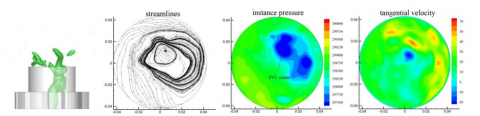## figure_10b.png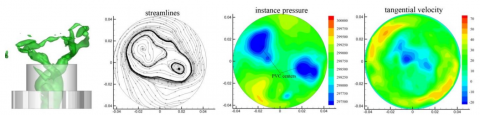## figure_10c.png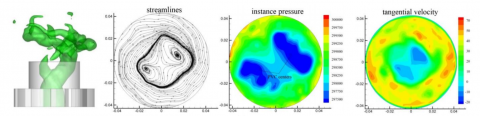Figure 10. Structure of the PVC visualized by a pressure iso-surface, streamlines, pressure and tangential velocity in the cross section of z=0.06 m in the non-reacting flow

## figure_11a.png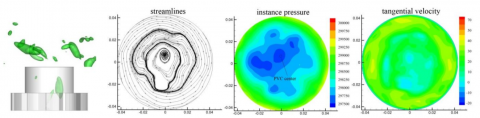## figure_11b.png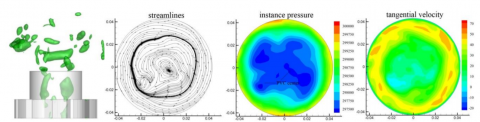## figure_11c.png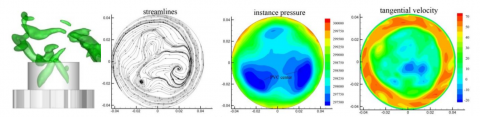Figure 11. Structure of the PVC visualized by a pressure iso-surface, streamlines, pressure and tangential velocity in the cross section of z=0.06 m in the reacting flow

## figure_12.png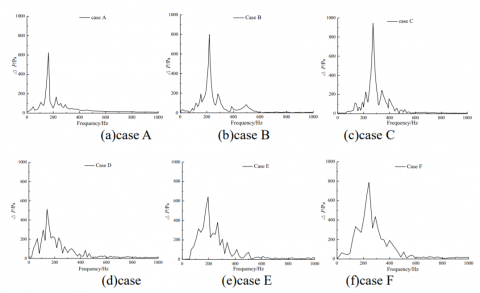Figure 12. Characteristics of the pressure oscillations inside the combustion chamber in both non-reacting and reacting flows

(Hz)

Due to the turbulent nature of the flame/flow interaction, the investigation of pressure oscillations in the non-reacting flow is a prerequisite for studying combustion instabilities. In the test rig, the periodic fluctuations of the reacting flow are captured by a piezo acoustic microphone installed in the combustion chamber . Figure 12 presents the Fourier transformed pressure spectra which is obtained at the same location as the test rig in both non-reacting and reacting flows during the caculation for all cases examined. It is clear that there is a linear increase in pressure oscillations amplitude with an increase in the inlet Reynold number in both non-reacting and reacting flows. The charactrisitcs of the effect of the inlet Reynold number on pressure oscillations amplitude is consistent with that of the studies of Kilian et al. . Furthermore, the amplitude of pressure oscillations in the non-reacting flow is relatively higher than that of the reacting flow for all cases examined. Comparing Figure 10 with Figure 11, it is clear that this happens because the pressure field in the non-reacting flow is dominated by the presence of the PVC which is suppressed in the reacting flow. The largest peaks in the spectrum of the pressure occasion occur at the frequencies of 163.9 Hz, 218.8 Hz and 272.9 Hz in cases A, B and C. The frequency of the PVC increases linearly with an increase in the inlet Reynold number of stream in the non-reacting flow. As aforementioned, the frequency of the PVC can be readily characterized by the non-dimensional parameter of Strouhal number $S_{t}=f \cdot D / u_{\infty}$. It is clear that the Strouhal number relatively remains constant which is approximately equal to 8.54 in cases A, B and C respectively. In case E, the dominant frequency is approximately 194.4 Hz which is in agreement with the experiment results in the studies of Stopper . It is clear that the effect of the inlet Reynold number on pressure occasion frequencies is similar to that in the non-reacting flow. There is also an anticipated decrease in frequency in the pressure frequency in the reacting flow. The Strouhal number decreases to about 7.54 in the reacting flow.

4. Conclusions

The effects of inlet Reynold number on the recirculation zone and precessing vortex core (PVC) behavior in both non-reacting and reacting flows are numerically studied by using a three-dimensional compressible three-dementional large eddy simulation approach. The predicted results show that a central toroidal recirculation zone (CTRZ) extends to the pilot surface of the combustor which could establish a firm aerodynamic base for ignition and combustion of fuel gas in the reacting flow. Under combustion conditions, the flow field became more complex and the structure of CTRZ is more irregular compared to that of the non-reacting flow, especially downstream of the flowfield. Furthermore, the PVCs with a helical structure formed upstream of CTRZ which precesses in the same direction as the global rotation of the flow and then compresses into a smaller vortex as it moves downstream in both non-reacting and reacting flows. Increasing the inlet Reynold number enlarges the magnitude of CTRZ and thus produces a more regular and stronger structure of the PVC inside the combustion chamber. Therefore, the breakdown positon of the PVC moves toward downstream of CTRZ with higher inlet Reynold numbers. The structure of the PVCs in the reacting flow mostly located in the regions of unburned reactants within the per-chamber which is relatively smaller and more irregular compared to that of the non-reacting flow. There is an increase in pressure oscillations amplitude and frequency with the increase in the inlet Reynold number. However, the Strouhal number remains relatively constant in the non-reacting flow. There is also an anticipated decrease in pressure oscillations, frequency and Strouhal number due to the compressibility effects of the flame in the reacting flow.

Acknowledgment

Project Supported by Fundamental Research Funds for the Central Universities (No. 2014ZZD04 and 2014XS17) and Beijing Natural Science Foundation (No. 3162030).

Nomenclature
 CFD Computational Fluid Dynamics CTRZ Central Toroidal Recirculation Zones DNS RANS Direct Numerical Simulations thermal Reynolds Average Navier-Stokes LES ORZ PVC Large Eddy Simulations Outer Recirculation Zones Precessing Vortex Core
References

 Huang Ying and V Yang, “Dynamics and stability of lean-premixed swirl-stabilized combustion,” Progress in Energy and Combustion Science, vol. 35, no. 4, pp. 293-364, 2009. DOI:10.1016/j.pecs.2009.01.002.

 N Syred, “A review of oscillation mechanisms and the role of the precessing vortex core (PVC) in swirl combustion systems,” Progress in Energy & Combustion Science, vol. 32, no. 2, pp. 93–161, 2006. DOI: 10.1016/j.pecs. 2005.10.002.

 Liu Y and Tang H, “CFD simulations of turbulent flows in a twin swirl combustor by RANS and hybrid RANS/LES methods,” Energy Procedia, vol. 66, pp. 329-332, 2015. DOI: 10.1016/j.egypro.2015. 02.078.

 F. Biagioli and F. Güthe, “Combustion dynamics linked to flame behaviour in a partially premixed swirled industrial burner,” Experimental Thermal & Fluid Science, vol. 32, no.7, pp. 1344-1353, 2007. DOI: 10.1016/j.expthermflusci.2007.11.007.

 Leibovich S, “Vortex stability and breakdown: Survey and extension,” AIAA Journal, vol. 22, no. 9, pp. 1192-1206, 1984. DOI:10.2514/3.8761.

 Faler Jh, “An experimental map of the internal structure of a vortex breakdown,” Journal of Fluid Mechanics, vol. 86, pp. 313-335, 1977. DOI: http://dx. doi. org /10.1017/S0022112078001159.

 S. T, “On stationary and travelling vortex breakdowns,” Journal of Fluid Mechanics, vol. 45, no.3, pp. 545-559, 1971. DOI:  http://dx.doi.org/10. 1017/S002211207100 0181.

 S. T, “Vortex Breakdown in Swirling Conical Flows,” AIAA Journal, vol. 9, pp. 1792-1799, 1971. DOI:  http: //dx.doi.org/10.2514/3.49981.

 W. J. Grabowski and S. A. Berger, “Solutions of the Navier-Stokes equations for vortex breakdown,” Journal of Fluid Mechanics, vol. 75, no. 3, pp. 525-544, 1976. DOI: http://dx.doi.org/10.1017/S00221120 76000360.

 S. RE, “Transition from spiral- to bubble-type vortex breakdown,” Physics of Fluids, vol. 8, no.5, pp. 1330-1332, 1996. DOI: http://dx.doi.org/10.1063/1.868902.

 Eldrainy Y. A. and Saqr K. M., “CFD insight of the flow dynamics in a novel swirler for gas turbine combustors,” International Communications in Heat & Mass Transfer, vol. 36, no. 9, pp. 936–941, 2009. DOI: 10.1016/j.icheatmasstransfer.2009.06.013.

 Guo B. and Fletcher D., “RANS calculations and measurements of instabilities in swirl-stabilised jets and flames,” Australian Symp on Combustion & Australian Flame Days, pp. 585-596, 2005. DOI: 0-7326-2251-4 (2003).

 K. Oberleithner and M. Stöhr, “Formation and flame-induced suppression of the precessing vortex core in a swirl combustor: Experiments and linear stability analysis,” Combustion and Flame, vol. 162, pp. 3100-3114, 2015. DOI: 10.1016/j. combustflame. 2015. 02. 015.

 Guo Hongtao and Li Guoshuai, “Numerical simualtion research on the transonic aeroelasticity of a high-aspect-ratio wing,” Int J of Heat & Tech, vol. 33, pp. 4, pp. 173-180, 2015. DOI: 10.18280/ijht.330422.

 Linlin Liu and Chuliang Wan, “CFD simulation and structuer optimization of the hot-air drying oven of a gravuer printing machine,” Int J of Heat & Tech, vol. 33, pp. 4, pp. 197-202, 2015. DOI: 10.18280/ijht. 330426.

 Bulat G., “prediction of aerodynamic frequencies in a gas trubine combustor using transient CFD,” in Proc. the ASME Turbo Expo, pp. 585-596, 2009. DOI: 10.1115/GT2009-59721.

 Syred N. and Beér J. M., “Combustion in swirling flows: a review,” Combustion and Flame, vol. 23, no. 2, pp. 143-201, 1974. DOI: 10.1016/0010-2180(74) 90057-1.

 K. Cui and B. Liu, “Numerical simulation of oxy-coal combustion for a swirl nurner with EDC model,” Chinese Journal of Chemical Engineering, vol. 22, no. 2, pp. 193-201, 2014. DOI: 10.1016/S1004-9541 (14) 60032-X.

 G. C. Krieger and A.P.V. Campos, “Numerical simulation of oxy-fuel combustion for gas turbine applications,” Applied Thermal Engineering, vol. 78, pp. 471-481, 2015. DOI: 10.1016/j.applthermaleng.20 15. 01.001.

 G. Bulat and E. Fedina, “Reacting flow in an industrial gas turbine combustor: LES and experimental analysis,”Proceedings of the Combustion Institute, vol. 35, no. 3, pp. 3175-3183, 2015. DOI: 10.1016/j.proci. 2014.05.015.

 S. Tanaka and M. Shimura, “DNS of turbulent swirling premixed flame in a micro gas turbine combustor,” Proceedings of the Combustion Institute, vol. 33, no. 2, pp. 3293-3300,2011.DOI:10.1016/j.proci.2010.07.034.

 G. Boudier and L.Y.M., “Comparison of LES, RANS and experiments in an aeronautical gas turbine combustion chamber,” Proceedings of the Combustion Institute, vol. 31, no. 2, pp. 3075-3082, 2007. DOI:10.1016/j. proci.2006.07.067.

 Carla Balocco and Giuseppe Petrone, “Thermo-fluid dynamics analysis and air quality for different ventilation patterns in an operating theater,” Int J of Heat & Tech, vol. 33, no. 4, pp. 25-32, 2015. DOI: 10.18280/ijht.330404.

 G. Bulat and W.P. Jone, “Large Eddy Simulation of an industrial gas-turbine combustion chamber using the sub-grid PDF method,” Proceedings of the Combustion Institute, vol. 34, no. 2, pp. 3155-3164, 2013. DOI: 10.1016/j.proci.2012.07.031.

 SHI Li and FU Zhongguang, “LES of swirl angle on combustion dynamic and NOx formation in a hybrid industrial combustor,” Int J of Heat & Tech, vol. 34, no. 2, pp. 197-206, 2016. DOI: 10.18280/ijht.340207.

 U. Stopper and M. Aigner, “PIV, 2D-LIF and 1D-Raman measurements of flow field, composition and temperature in premixed gas turbine flames,” Experimental Thermal and Fluid Science, vol. 34, no. 3, pp. 396-403, 2010. DOI: 10.1016/j.expthermflusci. 2009.10. 012.

 G. Bulat and W.P. Jones, “NO and CO formation in an industrial gas-turbine combustion chamber using LES with the Eulerian sub-grid PDF method,” Combustion and Flame, vol. 161, no. 7, pp. 1804-1825, 2014. DOI: 10.1016/j.combustflame.2013.12.028.

 B. Franzelli and E. Ribe, “Large Eddy Simulation of combustion instabilities in a lean partially premixed swirled flame,” Combustion and Flame, vol. 159, no. 2, pp. 621-637, 2012. DOI:10.1016/j. combust flame.20 11.08.004.

 G. Bulat and W. P. Jones, “Large Eddy Simulations of isothermal confined swirling flow in an industrial gas-turbine,” International Journal of Heat and Fluid Flow, vol. 51, pp. 50-64, 2015. DOI: 10.1016/j.ijheat fluidflow.2014.10.028.

 L.Y.M. Gicquel and G. Staffelbach, “Large Eddy Simulations of gaseous flames in gas turbine combustion chambers,” Progress in Energy and Combustion Science, vol. 38, no. 6, pp. 782-817, 2012. DOI: 10.10 16/j. pecs.2012.04.004.

 L. Selle, “Compressible large eddy simulation of turbulent combustion in complex geometry on unstructured meshes,” Combustion and Flame, vol. 137, pp. 489-505, 2004. DOI: 10.1016/j.combust flam e. 2004.03.008.

 U. Stopper and W. Meie, “Experimental study of industrial gas turbine flames including quantification of pressure influence on flow field, fuel/air premixing and flame shape,” Combustion and Flame, vol. 160, pp. 2103-2118, 2013. DOI: 10.1016/j.combust flame. 2013. 04.005.